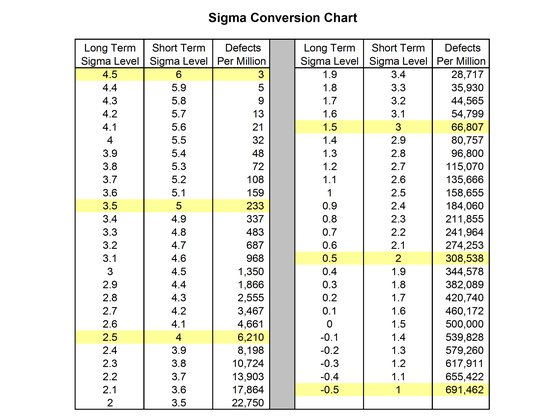# Sigma Conversion Chart

The chart below shows the theoretical defect rates that can be expected from various process sigma levels, assuming that the underlying data is normally distributed.

This chart can be used in a couple of different ways –

1. For continuous data collected over the short term, calculate the process sigma and find the value in the Short Term Sigma Level column. The theoretical defect level over the long run is noted in the Defects Per Million column. If data has been collected over sufficient time to include long term variation sources, use the Long Term Sigma Level column.
2. In cases where attribute data exists (typically in the form of % defective), it is sometimes desirable to know the equivalent process sigma for the process, even though the data may not follow a normal distribution. In these cases, convert the known defect rate into a defects-per-million value and find it on the chart.